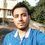# AMTI Practice corner !

I have heard about NMTC and AMTI , and this year I will be giving an olympiad for the first time . So let's have a AMTI practice corner, as the NMTC is 2 or 3 months from now.

Please contribute as many problems and their various solutions so as to help every other aspirant !!!

I am posting the first problem here .

###### AMTI, RMO, NMTC5 years, 3 months ago

This discussion board is a place to discuss our Daily Challenges and the math and science related to those challenges. Explanations are more than just a solution — they should explain the steps and thinking strategies that you used to obtain the solution. Comments should further the discussion of math and science.

When posting on Brilliant:

• Use the emojis to react to an explanation, whether you're congratulating a job well done , or just really confused .
• Ask specific questions about the challenge or the steps in somebody's explanation. Well-posed questions can add a lot to the discussion, but posting "I don't understand!" doesn't help anyone.
• Try to contribute something new to the discussion, whether it is an extension, generalization or other idea related to the challenge.

MarkdownAppears as
*italics* or _italics_ italics
**bold** or __bold__ bold
- bulleted- list
• bulleted
• list
1. numbered2. list
1. numbered
2. list
Note: you must add a full line of space before and after lists for them to show up correctly
paragraph 1paragraph 2

paragraph 1

paragraph 2

[example link](https://brilliant.org)example link
> This is a quote
This is a quote
    # I indented these lines
# 4 spaces, and now they show
# up as a code block.

print "hello world"
# I indented these lines
# 4 spaces, and now they show
# up as a code block.

print "hello world"
MathAppears as
Remember to wrap math in $$ ... $$ or $ ... $ to ensure proper formatting.
2 \times 3 $2 \times 3$
2^{34} $2^{34}$
a_{i-1} $a_{i-1}$
\frac{2}{3} $\frac{2}{3}$
\sqrt{2} $\sqrt{2}$
\sum_{i=1}^3 $\sum_{i=1}^3$
\sin \theta $\sin \theta$
\boxed{123} $\boxed{123}$

Sort by:

Problem 2 :

$I_n = \int \frac{cos(n\theta)}{cos\theta}$

$\text{Show that : } (n-1)(I_n+I_{n-2}) = 2sin(n-1)\theta$

- 5 years, 3 months ago

Great initiative! Do you know how to register for the exam and what are the list of test centers(I am from Bangalore)?

- 5 years, 3 months ago

Yeah. NMTC is the first step. Visit amtionline.com and check the toll free number there . the forms are available at the first week of June. It's an all India test and the centres will definitely include Bangalore

- 5 years, 3 months ago

thank you!

- 5 years, 3 months ago

Oh bdw check my note of online junior mathematician search. It's an o online contest. Lasts til 10th may. Join it plz

- 5 years, 3 months ago

I think that I already submitted the answers. I didn't get any message after the submission though.

- 5 years, 3 months ago

Yeah it must give u

- 5 years, 3 months ago

Oh when diu submit? Was it shown that your response has been recorded?

- 5 years, 3 months ago

Bdhoi amra pathaini ..otai bl6a

- 5 years, 3 months ago

Problem 1 :

Prove that there are infinitely many reals $p$ such that $p(p-3\text{{p}})$ is an integer where p is not an integer.

$\mathbf{Clarification : } \text{ {.} denotes fractional part}$

- 5 years, 3 months ago

Let $f(x) = x^2 - 3x\{x\}$.

Consider the interval $[n, n + 1]$ for integer n.
$f(n) = n^2$
$f(n + 1) = (n + 1)^2$

For $p \in [n, n + 1], f(p) = p^2 - 3pn$ is continuous, and hence takes every value between $f(n)$ and $f(n+1)$, by the intermediate value theorem.

But we can then find $p$ such that $f(p) = n^2 + 1$ or whatever integer between $n^2$ and $(n + 1)^2$.
And since there are infinitely many $n$, we are done.

- 5 years, 3 months ago

Absolutely ! this is the standard approach. Let me just share another view which specifies the integers also.

$f(p) = p(p-3\text{{p}}) = p([p] - 2\text{{p}})$

Since f(p) is an integer and product of two factors wher p isn't a integer. So we must have ([p] - 2{p}) a integer as two non-integers never multiply to be a integer.

$[p] \in \mathbb{I} \implies \text{2{p}} \in \mathbb{I}$

It's clear that {p} = 0.5 for 2{p} to be a integer .

2{p} = 1 .

So p = [p] + 0.5. we may write p as $\frac{\bar{xyz....5}}{10} = p$ as it's non-integer part is 0.5.

so f(p) = $\frac{\bar{xyz....5}}{10}([p]-1)\implies 10|([p]-1)$

So $[p] = 10k+1$ $k \in [0,\infty)$

So we have infinitely many integers p of the form $(p = [p] + 0.5 = 10k+1.5)$

- 5 years, 3 months ago

You may post the next problem.

- 5 years, 3 months ago